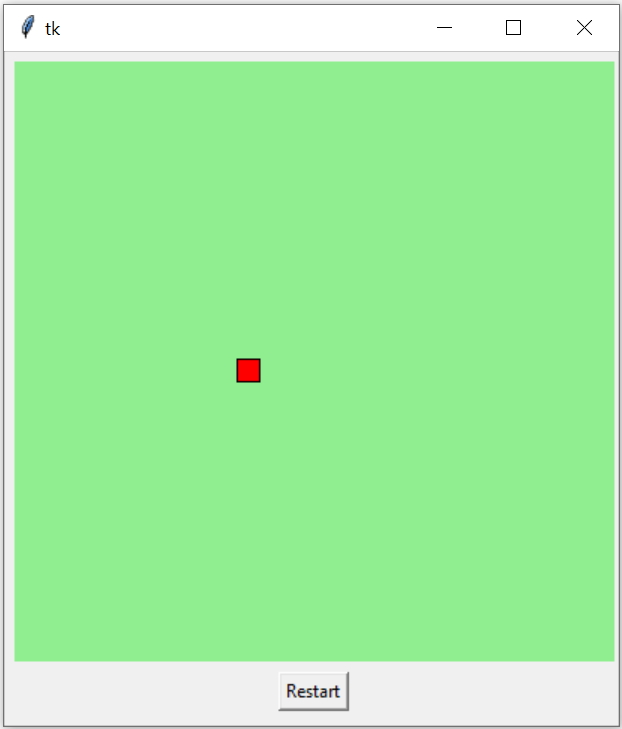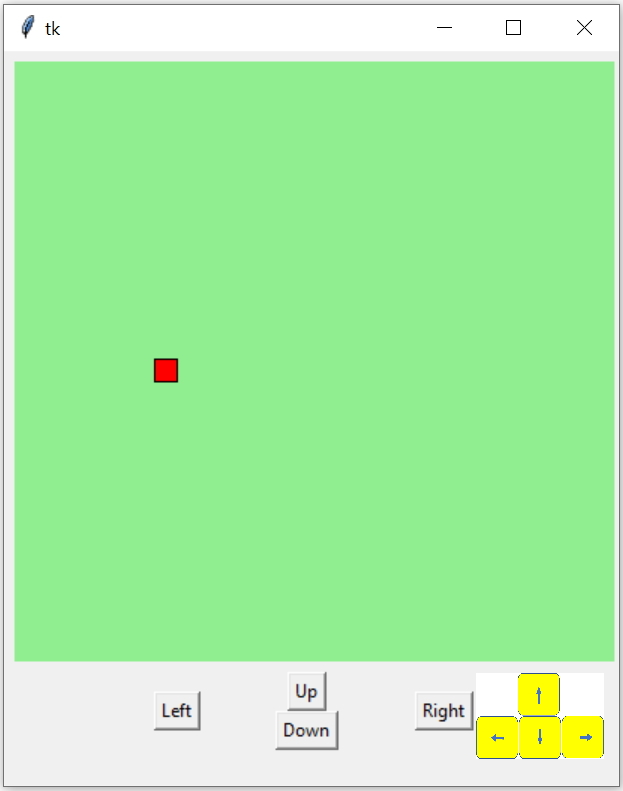# Movement of items in Canvas

Tkinter CanvasMoving objects in Tkinter Canvas in different directions by using buttons or by timer

## How to move any item on canvas ?

The basic concept of moving any item in canvas is to create , delete and then create the same item in new area by changing the coordinates.

## Moving a rectangle

For a rectangle we have two coordinates ( x1, y1 ) and (x2, y2) .

## Moving towards right side

While moving in horizontal direction (right side ) the coordinates for new locations will increase in x direction only. So the new location will be based on this formula. Here step is the variable whose value is the amount of jump or change the item will make.
``````x1=x1+step
x2=x2+step``````

## Moving towards left side

``````x1=x1-step
x2=x2-step``````
To move vertically we have to change the y coordinate value. To move in up direction we have to reduce the value.
``````y1=y1-step
y2=y2-step``````
To move in downward direction we have to increase the y coordinate values.
``````y1=y1+step
y2=y2+step ``````

## Making auto movement by using timer

We can repeat the movement by using timer after() , this will call the function after time delay in milliseconds.

Here time delay is 100 milliseconds and after the delay the function my_draw() is called.
``c1.after(100,my_draw)``

## How long the movement is allowed ?

We don’t want the coordinates to fall beyond the boundaries of the Canvas. So when the coordinates reaches the edge of the boundary and next movement ( based on the step value ) will take the element to outside the canvas, we have to stop there.
``````def my_draw():
global x1,y1,x2,y2,r1
c1.delete(r1) # delete the rectangle
r1=c1.create_rectangle(x1, y1, x2,y2,fill='red')
if (x2<(c_width-step)): # check for right edge
x1,x2=x1+step,x2+step # new coordinates of rectangle
c1.after(100,my_draw)  # recursive call after delay
else:
return   # stop recursive call and return to main ``````
Full code is here
``````import tkinter as tk
my_w = tk.Tk()
width,height=410,210 # set the variables
c_width,c_height=width-10,height-45 # canvas width height
d=str(width)+"x"+str(height)
my_w.geometry(d)
c1 = tk.Canvas(my_w, width=c_width, height=c_height,bg='lightgreen')

step=5 # value of each incremental movment, change this
x1,y1=5,int(c_height/2) # starting position
x2,y2=x1+15,y1+15      # starting position
r1=c1.create_rectangle(x1, y1, x2,y2,fill='red')  # draw rectangle

def my_draw():
global x1,y1,x2,y2,r1
c1.delete(r1) # delete the rectangle
r1=c1.create_rectangle(x1, y1, x2,y2,fill='red')
if (x2<(c_width-step)): # check for right edge
x1,x2=x1+step,x2+step # new coordinates of rectangle
c1.after(100,my_draw)  # recursive call after delay
else:
return   # stop recursive call and return to main

my_draw() # start moving, remove this if not required at beginning
def restart():
global x1,y1,x2,y2
x1,y1=5,int(c_height/2) # starting position
x2,y2=x1+15,y1+15      # starting position
my_draw() # start from starting position

b1=tk.Button(my_w,text='Restart',command=lambda:restart())
b1.grid(row=2,column=0)
my_w.mainloop()``````

## Using four directional buttons

In above code we have changed ( increased x or horizontal ) coordinates to move the rectangle in right direction.

Similarly we can decrease the x coordinates to move in left direction.
Increase the y coordinates to move in down direction.
Decrease the y coordinates to move in UP direction.

We will use four function to move the item in four different directions. We will add four buttons and each button will trigger the function based on the required direction. Inside the function we will control the coordinates to give the direction of movement to the element.``````import tkinter as tk
my_w = tk.Tk()
width,height=610,410 # set the variables
c_width,c_height=width-10,height-65 # canvas width height
d=str(width)+"x"+str(height)
my_w.geometry(d)
c1 = tk.Canvas(my_w, width=c_width, height=c_height,bg='lightgreen')

step=10 # Jump in each movement, change this value
x1,y1,x2,y2=5,200,20,215  # initial locations of rectangle
r1=c1.create_rectangle(x1, y1, x2,y2,fill='red')

def right(event):
global x1,y1,x2,y2,r1
if (x2<(c_width-step)):  # check right edge
x1=x1+step # increase the horizontal coordinates
x2=x2+step
c1.delete(r1) # delete the rectangle
r1=c1.create_rectangle(x1, y1,x2,y2,fill='red')
def left(event):
global x1,y1,x2,y2,r1
if(x1 > step): # check left edge
x1=x1-step # decrease the horizontal coordiantes
x2=x2-step
c1.delete(r1) # delete the rectangle
r1=c1.create_rectangle(x1, y1,x2,y2,fill='red')
def up(event):
global x1,y1,x2,y2,r1
if(y1 > step): # check top edge
y1=y1-step # decrease the vertical coordinates
y2=y2-step
c1.delete(r1) # delete the rectangle
r1=c1.create_rectangle(x1, y1,x2,y2,fill='red')
def down(event):
global x1,y1,x2,y2,r1
if(y2 < c_height-step): # check bottom edge
y1=y1+step # increase the vertical coordinates
y2=y2+step
c1.delete(r1) # delete the rectangle
r1=c1.create_rectangle(x1, y1,x2,y2,fill='red')

b1=tk.Button(my_w,text='Left',command=lambda:left('x'))
b1.grid(row=1,column=0,rowspan=2,sticky='E')
b2=tk.Button(my_w,text='Up',command=lambda:up('x'))
b2.grid(row=1,column=1)
b3=tk.Button(my_w,text='Right',command=lambda:right('x'))
b3.grid(row=1,column=2,rowspan=2,sticky='W')
b4=tk.Button(my_w,text='Down',command=lambda:down('x'))
b4.grid(row=2,column=1)

my_w.mainloop()``````

## Using Arrow Keys

We can connect the movement of the item to four directional arrow keys of our keyboard. The button and the arrow key press both can work so we will modify the function to receive the event like this.
``````def down(event):    # function declaration
#change the button click event.
b4=tk.Button(my_w,text='Down',command=lambda:down('x'))``````
All four functions we have to change like above and the click event of all four buttons need to be changed.

We have to bind the four arrow keys like this.
``````my_w.bind('<Right>',right) # binding to right arrow key
my_w.bind('<Left>',left)   # left arrow key pressed
my_w.bind('<Up>',up)       # up arrow key
my_w.bind('<Down>',down)   # down arrow key ``````
The full code
``````import tkinter as tk
my_w = tk.Tk()
width,height=610,410 # set the variables
c_width,c_height=width-10,height-65 # canvas width height
d=str(width)+"x"+str(height)
my_w.geometry(d)
c1 = tk.Canvas(my_w, width=c_width, height=c_height,bg='lightgreen')

step=10 # Jump in each movement, change this value
x1,y1,x2,y2=5,200,20,215  # initial locations of rectangle
r1=c1.create_rectangle(x1, y1, x2,y2,fill='red')

def right(event):
global x1,y1,x2,y2,r1
if (x2<(c_width-step)):  # check right edge
x1=x1+step # increase the horizontal coordinates
x2=x2+step
c1.delete(r1) # delete the rectangle
r1=c1.create_rectangle(x1, y1,x2,y2,fill='red')
def left(event):
global x1,y1,x2,y2,r1
if(x1 > step): # check left edge
x1=x1-step # decrease the horizontal coordiantes
x2=x2-step
c1.delete(r1) # delete the rectangle
r1=c1.create_rectangle(x1, y1,x2,y2,fill='red')
def up(event):
global x1,y1,x2,y2,r1
if(y1 > step): # check top edge
y1=y1-step # decrease the vertical coordinates
y2=y2-step
c1.delete(r1) # delete the rectangle
r1=c1.create_rectangle(x1, y1,x2,y2,fill='red')
def down(event):
global x1,y1,x2,y2,r1
if(y2 < c_height-step): # check bottom edge
y1=y1+step # increase the vertical coordinates
y2=y2+step
c1.delete(r1) # delete the rectangle
r1=c1.create_rectangle(x1, y1,x2,y2,fill='red')

b1=tk.Button(my_w,text='Left',command=lambda:left('x'))
b1.grid(row=1,column=0,rowspan=2,sticky='E')
b2=tk.Button(my_w,text='Up',command=lambda:up('x'))
b2.grid(row=1,column=1)
b3=tk.Button(my_w,text='Right',command=lambda:right('x'))
b3.grid(row=1,column=2,rowspan=2,sticky='W')
b4=tk.Button(my_w,text='Down',command=lambda:down('x'))
b4.grid(row=2,column=1)

my_w.bind('<Right>',right)
my_w.bind('<Left>',left)
my_w.bind('<Up>',up)
my_w.bind('<Down>',down)
my_w.mainloop()``````

## Moving automatically in all directions

Instead of triggering the functions ( in different directions ) left(), right(), up() , down() by using buttons or arrow keys , we can use timer to trigger the functions. After reaching the edge in any direction we can call another direction function to move the item in other direction.

We are checking the edges by using one if condition, so once the edge is reached by using the else part we can call different function to change the direction.
``````import tkinter as tk
my_w = tk.Tk()
width,height=610,410 # set the variables
c_width,c_height=width-10,height-65 # canvas width height
d=str(width)+"x"+str(height)
my_w.geometry(d)
c1 = tk.Canvas(my_w, width=c_width, height=c_height,bg='lightgreen')

step=10 # jump value
speed=100 # delay in milli seconds , decrease the value to increase speed
x1,y1=5,200
x2,y2=x1+15,y1+15
r1=c1.create_rectangle(x1,y1,x2,y2,fill='red')

def right():
global x1,y1,x2,y2,r1
if (x2<(c_width-step)): # check the right edge
c1.delete(r1) # delete the rectangle
x1,x2=x1+step,x2+step # increase the horizontal coordinates
r1=c1.create_rectangle(x1, y1,x2,y2,fill='red')
c1.after(speed,right)
else:
down()

def left():
global x1,y1,x2,y2,r1
if(x1>step): # check left edge
c1.delete(r1)
x1,x2=x1-step,x2-step
r1=c1.create_rectangle(x1,y1,x2,y2,fill='red')
c1.after(speed,left)
else: # edge is reached so change direction
up()
def up():
global x1,y1,x2,y2,r1
if(y1>step): # check top edge
c1.delete(r1)
y1,y2=y1-step,y2-step
r1=c1.create_rectangle(x1,y1,x2,y2,fill='red')
c1.after(speed,up)
else: # edge is reached so change direction
right()
def down():
global x1,y1,x2,y2,r1
if(y2 < (c_height-step)): # check bottom edge
c1.delete(r1)
y1,y2=y1+step,y2+step
r1=c1.create_rectangle(x1,y1,x2,y2,fill='red')
c1.after(speed,down)
else: # edge is reached so move left
left()

b1=tk.Button(my_w,text='Left',command=lambda:left())
b1.grid(row=1,column=0,rowspan=2,sticky='E')
b2=tk.Button(my_w,text='Up',command=lambda:up())
b2.grid(row=1,column=1)
b3=tk.Button(my_w,text='Right',command=lambda:right())
b3.grid(row=1,column=2,rowspan=2,sticky='W')
b4=tk.Button(my_w,text='Down',command=lambda:down())
b4.grid(row=2,column=1)

my_w.mainloop()``````

Subscribe to our YouTube Channel here

## Subscribe

* indicates required
Subscribe to plus2netplus2net.com

Post your comments , suggestion , error , requirements etc here